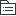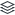货币时间价值

|
2

相关模板推荐

•第一节货币 时间价值 —— 作品大纲

•货币时间价 值的含义
• 在没有风险和没有通货膨胀的情况下，货币经历一定时间的投资和再投资所增加的价值，也称为资金的时间价值。(也称为资金的时间价值)
• 用相对数表示的货币的时间价值也称为纯粹利率（纯利率）。纯粹利率是指没有风险也没有通货膨胀情况下的社会平均利润率。
• 没有通货膨胀时，短期国库劵的利率可以视为纯利率
•复利终值 和现值
• 利息的两种计算方法
• 单利计息：只对本金计算利息，各期利息相等。
• 复利计息：既对本金计算利息，也对前期的利息计算利息，各期利息不同。
• 复利终值
• 现在的一定本金在将来一定时间，按复利计算的本金与利息之和，（在未来某个时间点的价值）简称本利和
• P=现值（初始值） F=终值（本利和） i=利率 n=计息期数
• 公式：F=P × （1+i）n =P×（F/P，i，n） 符号（F/P，i，n） （1+i）n 称为复利终值系数
• 年内计息多次：基本公式不变，只不过将年利率调为期利率（r/m），将年数调为期数。r=利率 m=一年计息次数 直接用m×年数。例：年利率4%，半年计息一次，一年就2次，4%/2 2×1=2
• 复利现值
• 指未来某时点的特定资金按复利计算，折算到现在的价值或者为取的将来一定的本利和，现在需要多少本金
• 与复利终值互为倒数 已知F、i、n，求P
• 公式：P=F / （1+i）-n =F × （1+i）-n=F×（P/F，i，n） 符号（P/F，i，n） （1+i）-n称为复利现值系数
•现值
• 普通年金现值
• 年金（A）：间隔期相等，每期金额相等。例：分期付款赊购、分期还贷、发放养老金等
• 第一期期末等额收付往前折算到0时点是多少钱，
• 公式：P=A×（P/A，i，n）
• 普通年金终值
• 第一期期末等额收付往后折（往后看）是多少钱
• 公式：F=A×（F/A，i，n）
• 预付年金现值
• 从第一期开始每期期初收款或付款的年金
• 公式：P=A×（P/A，i，n）×（1+i）或=A+A×（P/A，i，n-1）
• 预付年金终值
• 从第一期开始每期期初收款或付款的年金
• 公式：F=A×（F/A，i，n）×（1+i）或=A×（F/A，i，n-1）+A
• 递延年金现值
• 相对于普通年金的基础上，在第二期或第二期以后收付的年金。
• 递延期（m）：前若干期没有收支的期限，连续收支期（n）：A的个数
• 公式：P=A×（P/A，i，n）n是A的个数
• 递延年金终值
• 相对于普通年金的基础上，在第二期或第二期以后收付的年金。
• 公式：F=A×（F/A，i，n）n是A的个数
• 永续年金
• 无限期的普通年金。没有终值 公式：P=A/i
•年偿债基金和 年资本回收额
• 年偿债基金：已知F（终值），求A（年金） 公式：A=F/（F/A，i，n）
• 年资本回收额：已知P（现值），求A（年金） 公式：A=P/（P/A，i，n）
•利率计息
• 求 i。 计算方法：查表，如果无法查到则使用内插法，找系数表中相邻的系数。
• 现值或终值系数为止的利率计算
• 有时会出现一个表达式中含有两种系数，需逐步测试结合内插法，经过多次测试才能确定相邻的利率
• 例：已知5×(P/A，i,10)+100×(P/F，i,10)=104，求i的数值。
•名义利率与实际利率
• 一年多次计息时，给出的年利率是名义利率。—年计息多次时的利率和一年计息一次的利率是不一样的，因为存在时间价值。要使一年计息一次的利率=一年计息多次的利率，所以要求实际利率
• i 为实际利率、r为名义利率、m为每年复利计息的次数
• 周期利率=名义利率/年内计息次数=r/m
• 公式：实际利率 i =[1+(r/m)m-1] 1+i=(1+r/m)m
• 当每年计息一次时：实际利率=名义利率、当每年计息多次时：实际利率>名义利率
•通货膨胀情况下的名义利率与实际利率
• 名义利率中包含通货膨胀率。实际利率：是指剔除通货膨胀率后储户或投资者得到利息回报的真实利率。
• 1+名义利率=（1+实际利率）×（1+通货膨胀率）
• 实际利率=（1+名义利率）/（1+通货膨胀率）-1